# CVXGEN: Code Generation for Convex Optimization

## Example: Model Predictive Control (MPC)

This example, from control systems, shows a typical model predictive control problem. See the paper by Mattingley, Wang and Boyd for some detailed examples of MPC with CVXGEN.

## Optimization problem

We will model the optimization problemwith optimization variables

•(state variables)

•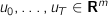(input variables)

and parameters

•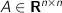(dynamics matrix)

•(transfer matrix)

•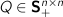(state cost)

•(final state cost)

•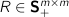(input cost)

•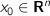(initial state)

•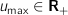(amplitude limit)

•(slew rate limit)

## CVXGEN code

dimensions
m = 2  # inputs.
n = 5  # states.
T = 10  # horizon.
end

parameters
A (n,n)  # dynamics matrix.
B (n,m)  # transfer matrix.
Q (n,n) psd  # state cost.
Q_final (n,n) psd  # final state cost.
R (m,m) psd  # input cost.
x (n)  # initial state.
u_max nonnegative  # amplitude limit.
S nonnegative  # slew rate limit.
end

variables
x[t] (n), t=1..T+1  # state.
u[t] (m), t=0..T  # input.
end

minimize